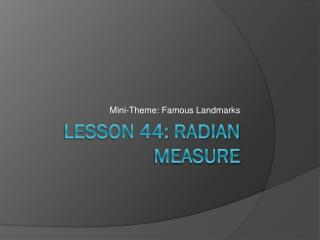DownloadDownload PresentationTélécharger la présentation- - - - - - - - - - - - - - - - - - - - - - - - - - - E N D - - - - - - - - - - - - - - - - - - - - - - - - - - -
##### Presentation Transcript

1. Mini-Theme: Famous Landmarks Lesson 44: Radian Measure

2. Follow up on Warm Up: • For #3 Assume that the hypotenuse of the triangle is the terminal ray of an angle in standard position that is 35 degrees. • Name two other co-terminal angles with this angle.

3. Complements/Supplements • Complementary angles sum to 90. • Supplementary angles sum to 180. • Examples:

4. Where is this landmark located?

5. Why is radian measure superior to degree measure? • The fact that there are 360 degrees in a circle is just based on some made up number. • Why do you think they chose 360 degrees? • Radians is based on an actual measurable quantity that is true of every circle. • Based on the ratio of pi.

7. Special Case

8. Examples:

9. Where is this located? Not to be confused with the space needle